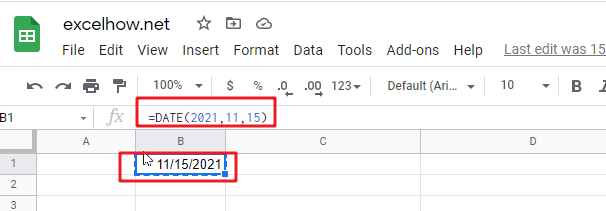# ExcelHow

This post will guide you how to use Google Sheets DATE function with syntax and examples.

## Description

The Google Sheets DATE converts a year, month, and day into a date.

The DATE function can be used to create a valid date from individual year, month and day value in google sheets. The purpose of this function is to create a date with year, month and day and its returned values is a valid date.

The DATE function is a build-in function in Google Sheets and it is categorized as a Date function.

## Syntax

The syntax of the DATE function is as below:

= DATE (year, month, day)

Where the VALUE function argument is:

• Text -This is a required argument. The year component of the date
• Month – This is a required argument. The month component of the date
• Day – This is a required argument. The day component of the date

Note:

• If the year value is between 0 and 1899, google sheets will add that value to 1900 to determine the year.
• If the year value is between 1900 and 9999, google sheets will use that value as the year value.
• If year is less than 0 or is greater than 9999, google sheets will return the error value: #NUM!
• If month is greater than 12, every 12 months will add 1 year to the year value.
• If the day value is greater than the number of days in the month specified, then it will add that number of days to the first day in the month. For example: DATA(2017,6,32), the DATE function will return “7/2/2017”

## Google Sheets DATE Function Examples

The below examples will show you how to use google sheets DATE Function to create a date.

#1 You can use the DATE function to create the dates with hard-coded numbers, just do the following formula:

`=DATE(2021,11,15) //it returns “11/15/2021”`